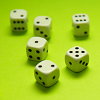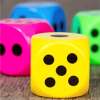#### You may also like### Roll These Dice

Roll two red dice and a green dice. Add the two numbers on the red dice and take away the number on the green. What are all the different possible answers?### Stop or Dare

All you need for this game is a pack of cards. While you play the game, think about strategies that will increase your chances of winning.### You Never Get a Six

Charlie thinks that a six comes up less often than the other numbers on the dice. Have a look at the results of the test his class did to see if he was right.

# Tricky Track

##### Age 7 to 11 Challenge Level:

To figure out the counter that wins most often, we first need to figure out the most common dice rolls.
There are $36$ different possible combinations. Here they are, in the format "First Die Roll - Second Die Roll - Total":
$1 - 1 - 2$
$1 - 2 - 3$
$1 - 3 - 4$
$1 - 4 - 5$
$1 - 5 - 6$
$1 - 6 - 7$
$2 - 1 - 3$
$2 - 2 - 4$
$2 - 3 - 5$
$2 - 4 - 6$
$2 - 5 - 7$
$2 - 6 - 8$
$3 - 1 - 4$
$3 - 2 - 5$
$3 - 3 - 6$
$3 - 4 - 7$
$3 - 5 - 8$
$3 - 6 - 9$
$4 - 1 - 5$
$4 - 2 - 6$
$4 - 3 - 7$
$4 - 4 - 8$
$4 - 5 - 9$
$4 - 6 - 10$
$5 - 1 - 6$
$5 - 2 - 7$
$5 - 3 - 8$
$5 - 4 - 9$
$5 - 5 - 10$
$5 - 6 - 11$
$6 - 1 - 7$
$6 - 2 - 8$
$6 - 3 - 9$
$6 - 4 - 10$
$6 - 5 - 11$
$6 - 6 - 12$

Number of appearances of $2$: $1$
Number of appearances of $3$: $2$
Number of appearances of $4$: $3$
Number of appearances of $5$: $4$
Number of appearances of $6$: $5$
Number of appearances of $7$: $6$
Number of appearances of $8$: $5$
Number of appearances of $9$: $4$
Number of appearances of $10$: $3$
Number of appearances of $11$: $2$
Number of appearances of $12$: $1$

Looking at all of the totals, we can see that $2$ appears once, $3$ twice, $4$ thrice, and so on until we get to $7$. At this point, the number of appearances starts to decrease. $8$ appears five times, $9$ four, and $12$ only once. We can see that $7$ appears most often, and is therefore most likely to win the race.

But why does $7$ appear most often? It is because there is no result for the first die rolled that excludes it as a result. If you look back at the table, you can see that no matter what is rolled on the first die, seven still has a $1$ in $6$ chance of being the result. This is not true for any other number. And this is why $7$ is the most common result.

What if the dice had seven faces? $7$ would no longer be the most common number, as a roll of $7$ on the first die would eliminate it as a result. Instead, $8$ is the most common, for the reasons mentioned above. If the dice had eight faces, then $9$ would be the most common. There is a pattern here: if two dice are being rolled, the most common result can be determined by adding $1$ to the number of faces each die has.

Very well done, Jacob. Here is another way to display the possible totals when rolling two $1-6$ dice:

 1 2 3 4 5 6 1 2 3 4 5 6 7 2 3 4 5 6 7 8 3 4 5 6 7 8 9 4 5 6 7 8 9 10 5 6 7 8 9 10 11 6 7 8 9 10 11 12